## Labs

#### Department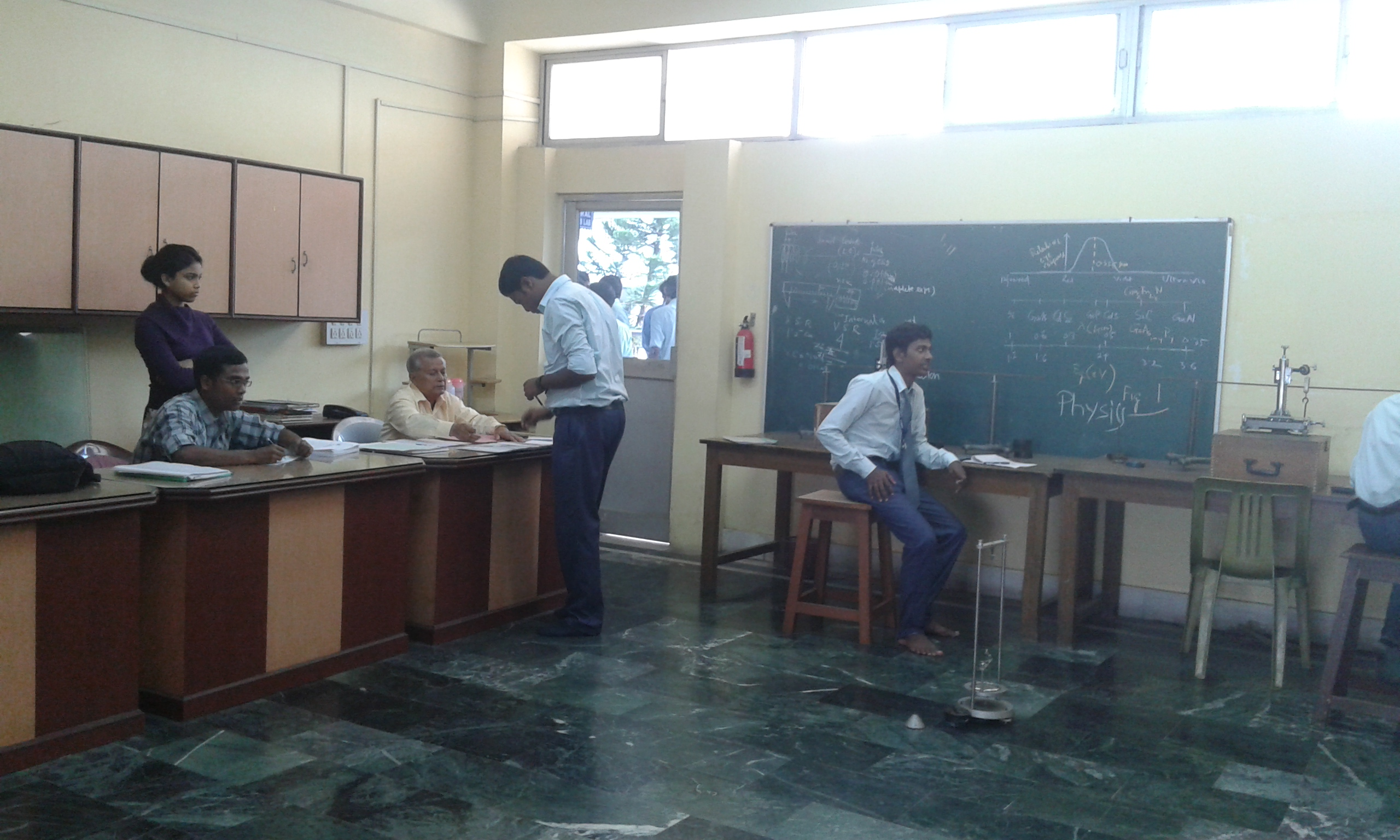#### General Physics lab & Classical Optics

##### Total area of the lab/workshop 1000 sqft

###### Name of experiment conducted
1. Study of torsional oscillation of torsional pendulum & determination of time period using various load of the oscillator.
2. To determine the Rigidity modulus of a Wire by static method.
3. Error analysis-EA1:Slider callipers & Screw gauge.
4. Error analysis-EA2 :Carey Foster Bridge.
5. Determination of wavelength of light by Newton's ring method.
6. Determination of wavelength of light by Fresnel's bi-prism method.
7. To determine the dispersive power of the given material of the prism.
8. Verification of Bohr's atomic orbital theory with the help of Frank-Hertz experiment.
9. Measurement of specific rotation of an optically active solution by polarimeter
10. Study of LCR circuit using ac signal and determination of Q factor.
11. Study of Hydrogen/ Helium spectrum using transmission grating and measurement of Rydberg Constant.
12. Measurement of nodal and antinodal points along a transmission wire and measurement of wave length.
13. To determine the thermal conductivity of a bad conductor by Lee's method.
14. To determine the thermal conductivity of a good conductor by Searle's method.
15. To determine the bending moment & shear force of beam by traveling microscope.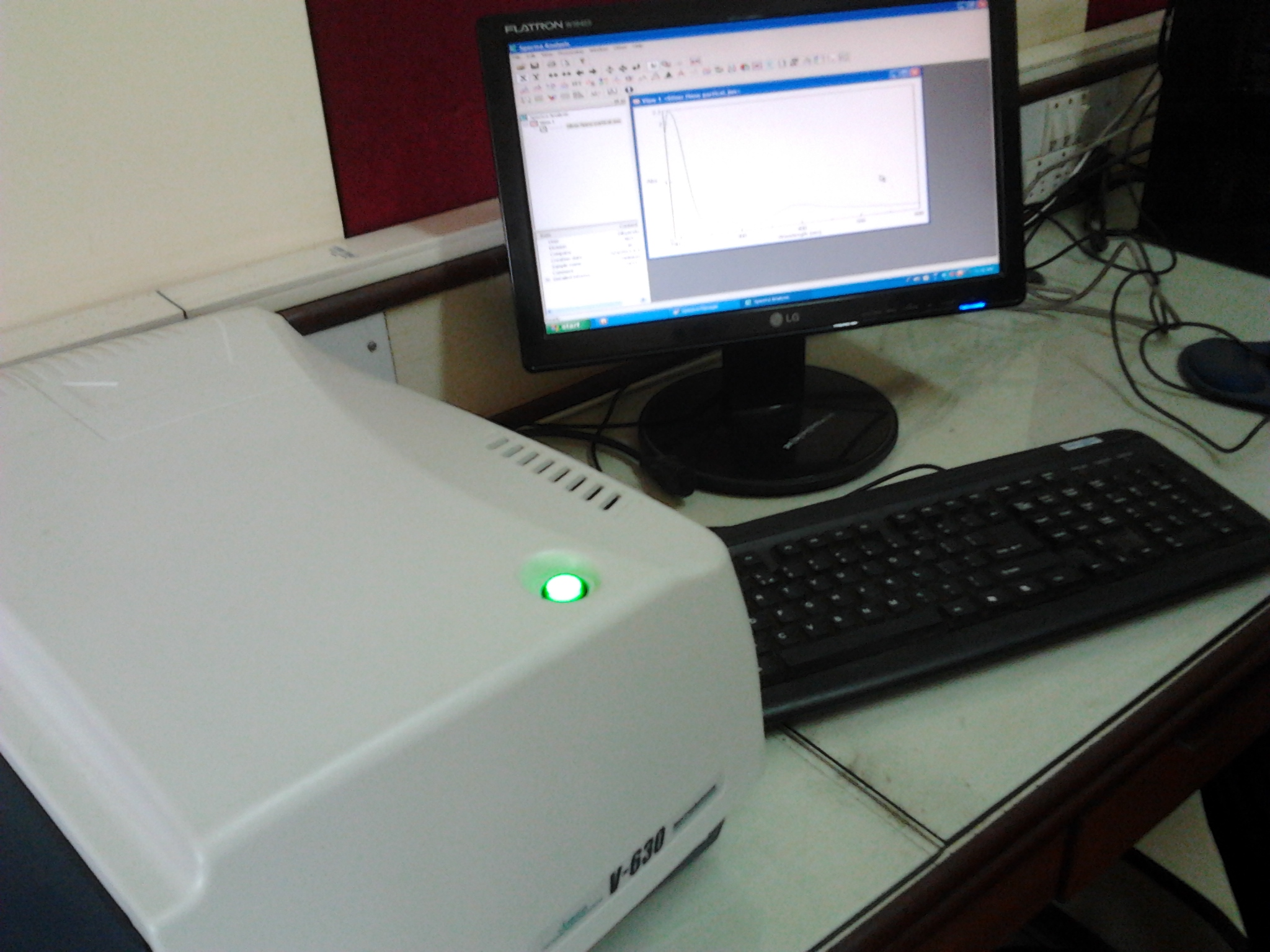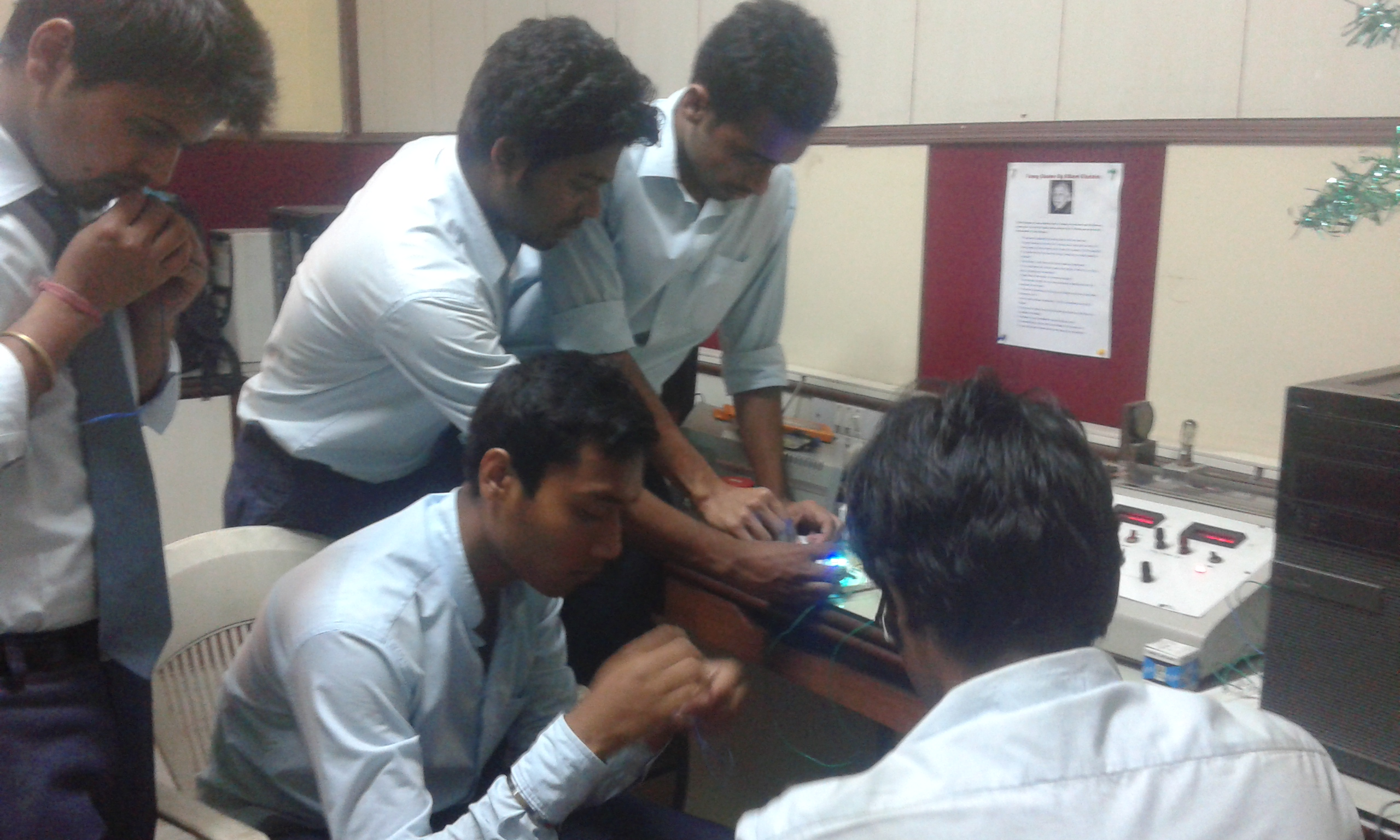#### Computational Physics & Modern Optics lab

##### Total area of the lab/workshop 1000 sqft

###### Name of experiment conducted
1. Determine of Wavelength of LASER with transmission grating
2. To determine numerical aperture and the energy losses related to optical fibre experiment
3. To study current-voltage characteristics, load response, areal characteristics and spectral response of photo voltaic solar cells & measurement of maximum workable power.
4. Phoenix tool kit designing science and engineering experiments using Computer Interface
5. Study of circularly and ellipticaly polarized light by using half-wave and quarter wave plates.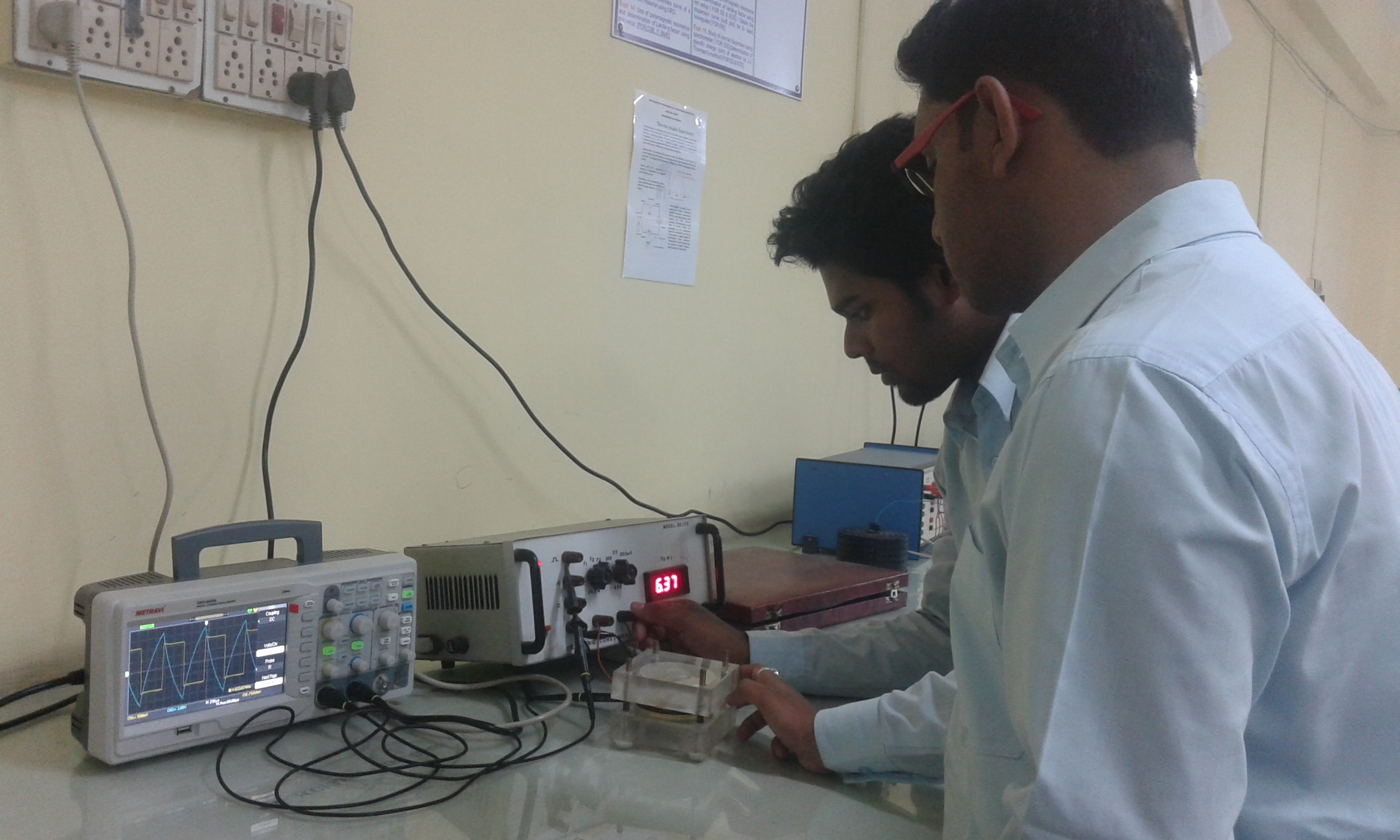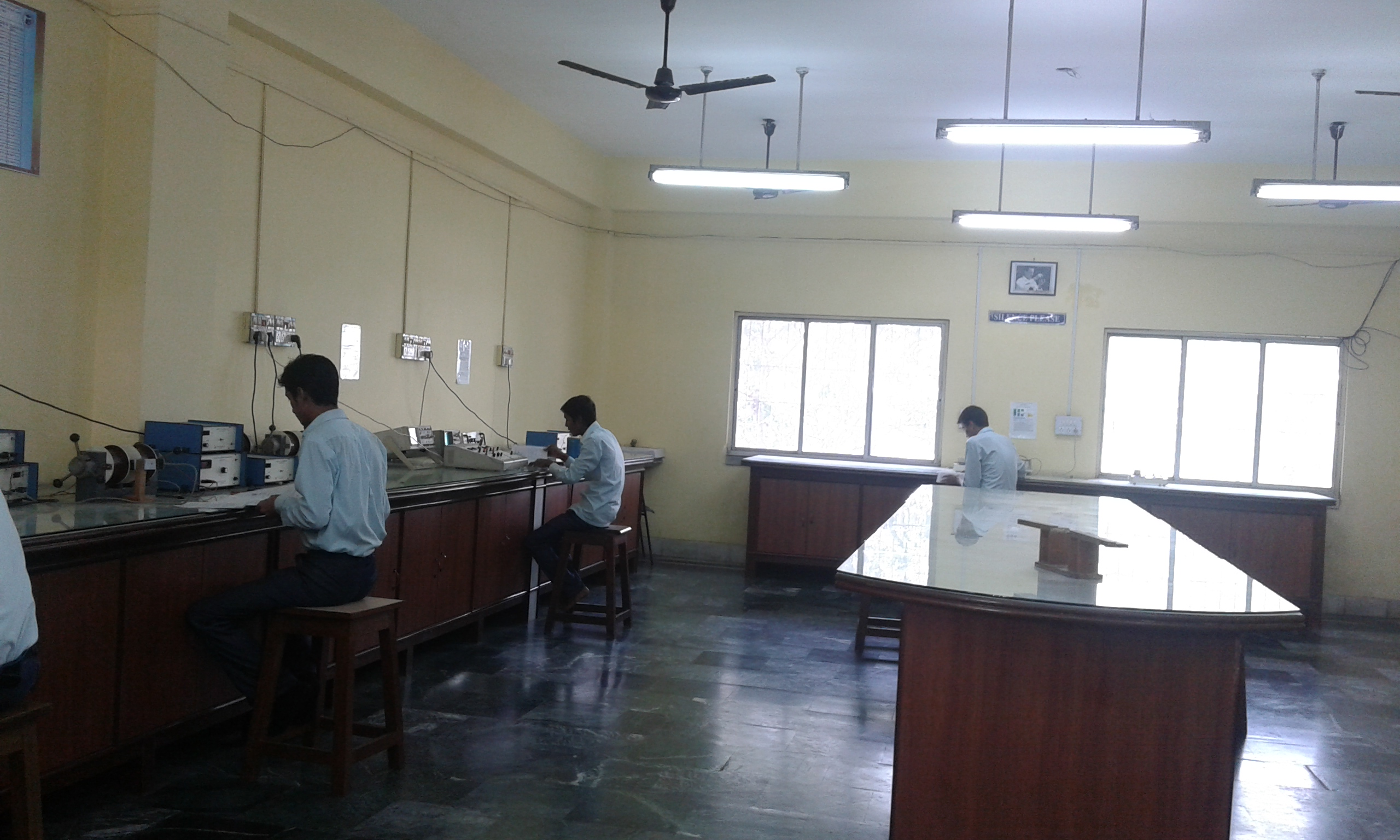#### Modern Physics lab

###### Name of experiment conducted
1. Determination of band gap of a semiconductor/thermistor
2. Determination of Hall co-efficient of a semiconductors [FOR CSE, IT,ECE, EIE,EE,BME]
3. Measurement of Magnetoresistance of a semiconductor
4. Determination of Stefan's radiation constant using the principle of pyrometer [FOR ME, CE]
5. Study of a temperature sensor characteristics/Study of transducer property: Determination of the thermo-electric power at a certain temperature of the given thermocouple.
6. Determination of Planck's constant using the principle of pyrrometer [FOR ME, CE]
7. Study of dielectric constant of different solids with the provision of frequency variation with CRO.
8. Measurement of losses in a dielectric using LCR ckt [FOR ME, CE].
9. Measurement of Curie temperature of the given sample Ferroelectrics Ceramics, Model: DEC-01
10. Determination of e/m by Thomson's method (bar magnet) (for BME, EE, ECE)
11. Study of dipolar magnetic field behavior. [FOR CSE, IT, ECE,EE,EIE]
12. Study of I-V characteristics of a LED [FOR CSE, IT,BME].
13. Study of I-V characteristics of a LDR [FOR CSE, IT,BME].
14. Determination of velocity of ultrasonic wave using piezoelectric crystal & compressibility of the given liquid.
15. Study of I-V characteristics of Tunnel diode. [FOR CSE, IT, ECE, EIE]
16. Study of hysteresis curve of a ferromagnetic material using CRO.
17. Use of paramagnetic resonance and determination of lande-g factor using esr setup. [FOR CSE, IT, BME,ECE,EE]
18. Study of drain characteristics and transfer characteristics of a MOSFET and hence determine the FET parameters (drain resistance, transconductance & amplification factor). [ FOR EE & EIE]
19. Study of lattice dyanamics and analysis of phonon modes in a crystal [FOR ME & CE]
20. Study of I-V characteristics of a Varactor diode [FOR ECE & EIE]
21. Obtain the dispersion curve (ω-β plot) for X- band waveguide. [FOR EIE]
22. Study of I-V characteristics ofPhtototransistor [FOR CSE,IT,ECE & EIE]
23. Study of I-V characteristics ofSCR [FOR CSE,IT,ECE & EIE]